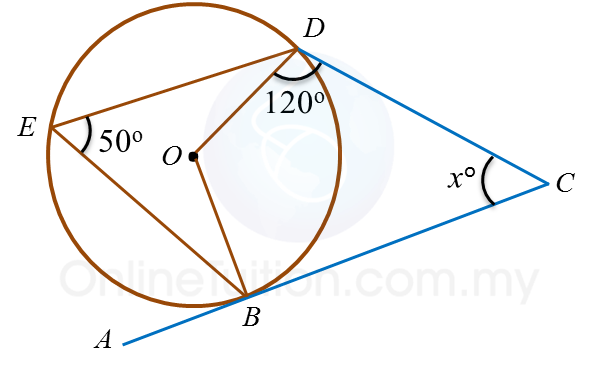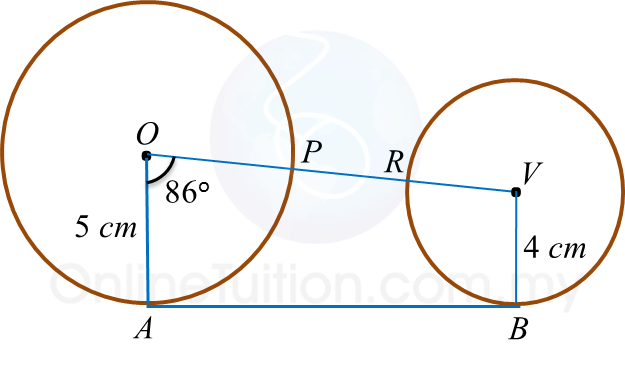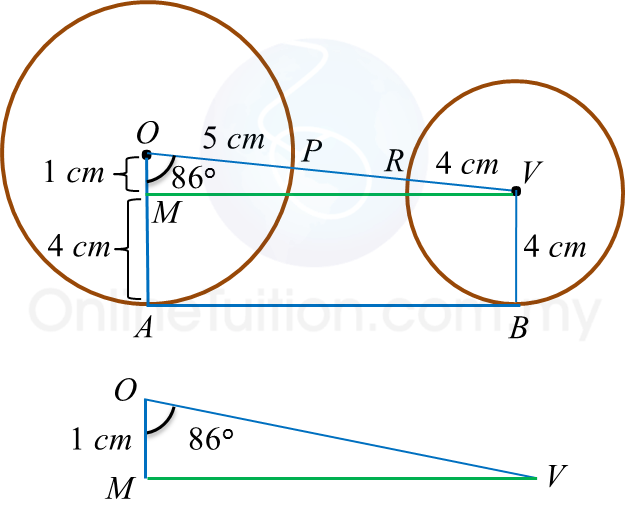# 6.7.4 Angles and Tangents of Circles, PT3 Focus Practice

Question 9:In figure above, ABC is a tangent to the circle centre O, at point B.
The value of is

Solution:
OBC = 90
BOD = 2 × 50o = 100o
x= 360– ∠BOD – ∠OBC – 120o
= 360– 100o – 90o – 120o
= 50

Question 10:The figure above shows two circles with respective centres O and V. AB is a common tangent to the circles. OPRV is a straight line. The length, in cm, of PR is

Solution:$\begin{array}{l}\mathrm{cos}{86}^{o}=\frac{OM}{OV}\\ 0.070=\frac{1}{OV}\\ OV=\frac{1}{0.070}\\ OV=14.29cm\\ \\ \therefore PR=14.29-5-4\\ \text{}=5.29cm\end{array}$